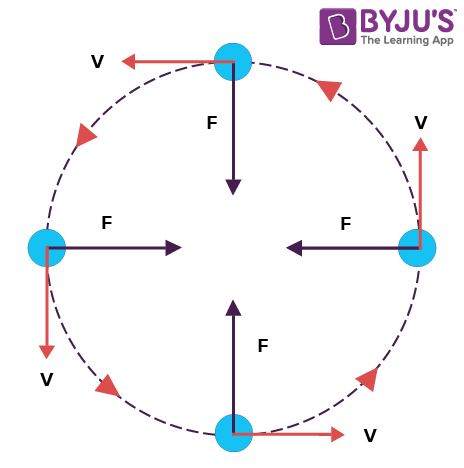# When a particle moves in a circle with a uniform speed (1) Its velocity and acceleration are both constant (2) Its velocity is constant but the acceleration changes (3) Its acceleration is constant but the velocity changes (4) Its velocity and acceleration both change.

Answer: (4) Its velocity and acceleration both change.

In uniform circular motion speed is constant, but the direction is changing at each point of motion. Hence velocity varies continously. Therefore, uniform circular motion is also known as uniform accelerated motion.

## Uniform Circular Motion

The movement of a body following a circular path is called a circular motion. Now, the motion of a body moving with constant speed along a circular path is called Uniform Circular Motion. Here, the speed is constant but the velocity changes.

.If a particle is moving in a uniform circular motion:

• Its speed is constant
• Velocity is changing at every instant
• There is no tangential acceleration
• Radial (centripetal) acceleration = ω2r
• v = ωr(0)(1)